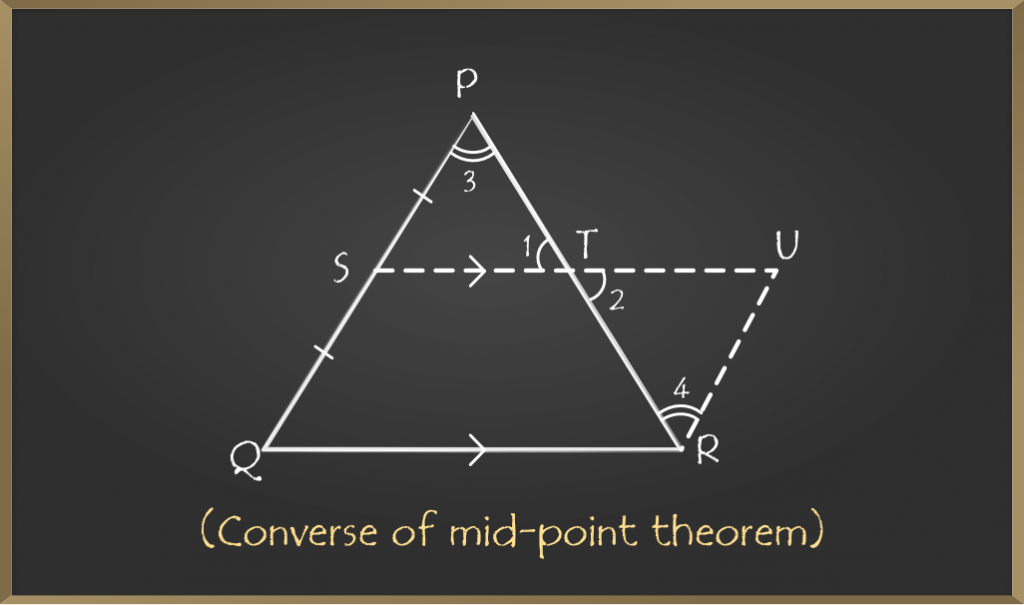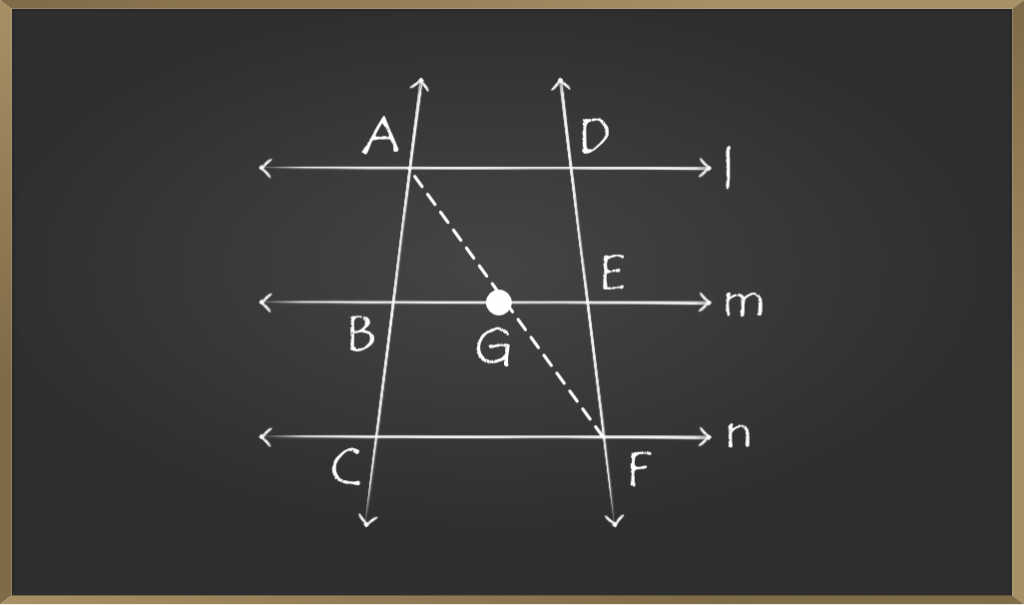# Mid Point Theorem – Quadrilaterals | Class 9 Maths

• Last Updated : 28 Oct, 2020

Geometry is an important part of mathematics that deals with different shapes and figures. Triangles are an important part of geometry and the mid-point theorem points towards mid points of the triangle.

## What is Mid-Point Theorem?

This theorem states that” The line segment joining mid-points of two sides of a triangle is parallel to the third side of the triangle and is half of it”

Attention reader! All those who say programming isn't for kids, just haven't met the right mentors yet. Join the  Demo Class for First Step to Coding Coursespecifically designed for students of class 8 to 12.

The students will get to learn more about the world of programming in these free classes which will definitely help them in making a wise career choice in the future.## Proof of Mid-Point Theorem

A triangle ABC in which D is the mid-point of AB and E is the mid-point of AC.

To Prove: DE ∥ BC and DE = 1/2(BC)

### Construction

Extend the line segment joining points D and E to F such that DE = EF and join CF.

### Proof

In ∆AED and ∆CEF

DE = EF (construction)

∠1 = ∠2 (vertically opposite angles)

AE = CE (E is the mid-point)

△AED ≅ △CEF by SAS criteria

Therefore,

∠3 =∠4 (c.p.c.t)

But these are alternate interior angles.

So, AB ∥ CF

But AD = DB (D is the mid-point)

Therefore, BD = CF

In BCFD

BD∥ CF (as AB ∥ CF)

BD = CF

BCFD is a parallelogram as one pair of opposite sides is parallel and equal.

Therefore,

DF∥ BC (opposite sides of parallelogram)

DF = BC (opposite sides of parallelogram)

As DF∥ BC, DE∥ BC and DF = BC

But DE = EF

So, DF = 2(DE)

2(DE) = BC

DE = 1/2(BC)

Hence, proved that the line joining mid-points of two sides of the triangle is parallel to the third side and is half of it.

## What is the Converse of Mid-Point Theorem?

The line drawn through the mid-point of one side of a triangle parallel to the base of a triangle bisects the third side of the triangle.## Proof of the Theorem

In triangle PQR, S is the mid-point of PQ and ST ∥ QR

To Prove: T is the mid-point of PR.

### Construction

Draw a line through R parallel to PQ and extend ST to U.

### Proof

ST∥ QR(given)

So, SU∥ QR

PQ∥ RU (construction)

Therefore, SURQ is a parallelogram.

SQ = RU(Opposite sides of parallelogram)

But SQ = PS (S is the mid-point of PQ)

Therefore, RU = PS

In △PST and △RUT

∠1 =∠2(vertically opposite angles)

∠3 =∠4(alternate angles)

PS = RU(proved above)

△PST ≅ △RUT by AAS criteria

Therefore, PT = RT

T is the mid-point of PR.

### Sample Problems on Mid Point Theorem

Problem 1: l, m, and n are three parallel lines. p and q are two transversals intersecting parallel lines at A, B, C, D, E, and F as shown in the figure.If AB:BC = 1:1, find the ratio of DE : EF.Given: AB:BC=1:1

To find: DE:EF

Construction: Join AF such that it intersects line m at G.

In △ACF

AB = BC(1:1 ratio)

BG∥ CF(as m∥n)

Therefore, by converse of mid-point theorem G is the midpoint of AF(AG = GF)

Now, in △AFD

AG = GF(proved above)

Therefore, by converse of mid-point theorem E is the mid-point of DF(FE = DE)

So, DE:EF = 1:1(as they are equal)

Problem 2: In the figure given below L, M and N are mid-points of side PQ, QR, and PR respectively of triangle PQR.

If PQ = 8cm, QR = 9cm and PR = 6cm. Find the perimeter of the triangle formed by joining L, M, and N.Solution: As L and N are mid-points

By mid-point theorem

LN ∥ QR and LN = 1/2 * (QR)

LN = 1/2 × 9 = 4.5cm

Similarly, LM = 1/2 * (PR) = 1/2×(6) = 3cm

Similarly, MN = 1/2 * (PQ) = 1/2 × (8) = 4cm

Therefore, the perimeter of △LMN is LM + MN + LN

= 3 + 4 + 4.5

= 11.5cm

Perimeter is 11.5cm

Result: Perimeter of the triangle formed by joining mid-points of the side of the triangle is half of the triangle.

My Personal Notes arrow_drop_up